Thank you for visiting nature.com. You are using a browser version with limited support for CSS. To obtain the best experience, we recommend you use a more up to date browser (or turn off compatibility mode in Internet Explorer). In the meantime, to ensure continued support, we are displaying the site without styles and JavaScript.

# Coupling of Higgs and Leggett modes in non-equilibrium superconductors

## Abstract

In equilibrium systems amplitude and phase collective modes are decoupled, as they are mutually orthogonal excitations. The direct detection of these Higgs and Leggett collective modes by linear-response measurements is not possible, because they do not couple directly to the electromagnetic field. In this work, using numerical exact simulations we show for the case of two-gap superconductors, that optical pump–probe experiments excite both Higgs and Leggett modes out of equilibrium. We find that this non-adiabatic excitation process introduces a strong interaction between the collective modes, which is absent in equilibrium. Moreover, we propose a type of pump–probe experiment, which allows to probe and coherently control the Higgs and Leggett modes, and thus the order parameter directly. These findings go beyond two-band superconductors and apply to general collective modes in quantum materials.

## Introduction

Collective excitation modes are a characteristic feature of symmetry-broken phases of matter. These collective excitations are due to amplitude and phase fluctuations of the order parameters, which are decoupled in equilibrium systems, as they represent mutually orthogonal excitations. The properties of collective modes are of fundamental interest, as they are a distinguishing feature of any symmetry-broken phase, such as superconductors, charge-density waves or antiferromagnets. For example, superconductors exhibit an amplitude Higgs mode and a phase mode, which are the radial and angular excitations in the Mexican-hat potential of the free energy. In two-band superconductors there exists in addition a Leggett phase mode1, which corresponds to collective fluctuations of the interband phase difference.

As we show in this work, out-of-equilibrium excitations of symmetry-broken phases lead to a direct coupling between phase and amplitude modes, an effect which is absent in equilibrium systems. Furthermore, we demonstrate that ultrafast pump–probe measurements allow to directly probe and coherently control collective excitation modes. Pump–probe measurements have recently become a key tool to probe the temporal dynamics and relaxation of quantum materials2,3,4,5,6,7,8,9,10,11,12,13. In particular, this technique has been used to measure the oscillations of the amplitude Higgs mode of the one-gap superconductor NbN. It has been shown, both theoretically14,15,16,17,18,19,20,21,22,23,24,25,26,27,28 and experimentally2,3,4, that a short intense laser pulse of length τ much shorter than the dynamical time scale of the superconductor τΔh/(2|Δ|) induces oscillations in the order parameter amplitude at the frequency ωH=2Δ/ħ, with Δ the asymptotic gap value.

Although non-equilibrium collective modes in conventional single-gap superconductors are well understood, the investigation of collective excitations in unconventional non-equilibrium superconductors with multiple gaps, such as MgB2 or iron pnictides, is still in its infancy29,30,31. These multicomponent superconductors have a particularly rich spectrum of collective excitations1,32,33. In this study, we simulate the pump–probe process in a two-gap superconductor using a semi-numerical approach based on the density-matrix formalism. This method is exact for mean-field Hamiltonians15,21, captures the coupling between the superconductor and the electromagnetic field of the pump laser at a microscopic level and allows for the calculation of the pump–probe conductivity, as measured in recent experiments2,3. Two-gap superconductors exhibit besides the amplitude Higgs34 and the phase modes35,36, also a Leggett mode1, which results from fluctuations of the relative phase of the two coupled gaps, that is, equal but opposite phase shifts of the two-order parameters, see Fig. 1b. In equilibrium superconductors, the Higgs and Leggett modes are decoupled, as they correspond to mutually orthogonal fluctuations. In contrast to the phase mode, both Higgs and Leggett modes are charge neutral and therefore do not couple to the electromagnetic field37. As a consequence, these excitations cannot be detected directly with standard linear-response-type measurements. Observation of these modes has only been possible in special circumstance, for example, when they couple to another order parameter, such as in charge density wave systems38,39,40,41.

Here we show that in a pump–probe experiment both Leggett and Higgs modes can be excited out of equilibrium, and directly observed as oscillations in the absorption spectra at their respective frequencies. We find that the non-adiabatic excitation process of the pump pulse induces an intricate coupling between the two charge-neutral modes, which pushes the frequency of the Leggett mode below the continuum of two-particle excitations. Moreover, the frequencies of the Leggett and Higgs modes and the coupling between them can be controlled by the fluence of the pump pulse. Hence, by adjusting the laser intensity the two modes can be brought into resonance, which greatly enhances their oscillatory signal in the pump–probe absorption spectra.

## Results

### Pump-excitation process

In pump–probe measurements, the pump laser pulse excites a high density of quasiparticles above the gap of the order parameter, thereby modifying the Mexican-hat potential of the free energy (Fig. 1). As a result, the amplitude of the order parameter decreases, reducing the minimum of the free energy. If the pump-pulse-induced changes in occur on a faster time scale than the intrinsic response time of the symmetry-broken state, the collective modes start to oscillate at their characteristic frequencies about the new free-energy minimum (see Fig. 1a). In this work we study this non-adiabatic excitation mechanism for two-band superconductors perturbed by a short and intense pump pulse.

The Hamiltonian describing the two-band superconductor coupled to the pump laser field is given by H=HBCS+Hlaser, with the two-band Bardeen-Cooper-Schrieffer (BCS) mean-field Hamiltonian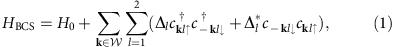where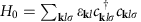denotes the normal state Hamiltonian and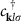creates electrons with momentum k, band index l and spin σ. The first sum in equation (1) is taken over the set W of momentum vectors with |ɛk l|≤ħωc=50 meV, ωc being the cutoff frequency. The gaps Δ1 and Δ2 in the two bands are determined at each temporal integration step from the BCS gap equations with the attractive intraband pairing interactions V1 and V2, and the interband coupling V12=V1. Motivated by the numbers for MgB2 (ref. 42), we fix V1 and V2 such that the gaps in the initial state take on the values Δ1(ti)=7 meV and Δ2(ti)=3 meV, and study the dynamics of the two-gap superconductor as a function of the relative interband coupling . Hlaser represents the interaction of the pump laser with the superconductor and contains terms linear and quadratic in the vector potential of the laser field, which is of Gaussian shape with central frequency ħω0=8 meV, pulse width τ=0.4 ps and light-field amplitude |A0|. We determine the dynamics of Hamiltonian (1) by means of the density matrix approach and solve the resulting equations of motion using Runge–Kutta integration (see Methods).

### Pump response

Pumping the two-band superconductor with a short laser pulse of length τ τΔ excites a non-thermal distribution of Bogoliubov quasiparticles above the gaps Δi, which in turn leads to a rapid, non-adiabatic change in the free-energy landscape (Fig. 1). As a result, the collective modes of the superconductor start to oscillate about the new minima of . This is clearly visible in Fig. 2, which shows the temporal evolution of the gap amplitudes |Δi| and of the phase difference Φ1–Φ2 between the two gaps. From the Fourier spectra in Figs 2d–f we can see that three different modes (and their higher harmonics) are excited at the frequencies ωH1, ωH2 and ωL. The two modes at ωH1 and ωH2 only exist in the dynamics of Δ1(t) and Δ2(t), respectively, and their peaks are located at the energy of the superconducting gaps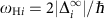, where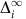denotes the asymptotic gap value14,15,16,17,18,19,20. This holds for all parameter regimes, even as the laser fluence is increased far beyond the linear absorption region (see Fig. 5). We therefore assign the peaks at ωH1 and ωH2 to the Higgs amplitude modes of the two gaps. The higher Higgs mode ωH1 is strongly damped, because it lies within the continuum of Bogoliubov quasiparticle excitations, which is lower bounded by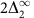. For the lower mode ωH2, on the other hand, the decay channel to quasiparticles is small, as ωH2 is at the continuum threshold. This is similar to the non-equilibrium Higgs mode of the single-gap superconductor NbN, whose oscillations have recently been observed over a time period of about 10 ps by pump–probe measurements2,3.

Interestingly, two-band superconductors exhibit a third collective mode besides the two Higgs modes at a frequency ωL below the quasiparticle continuum. This mode is most clearly visible in the dynamics of the phase difference Φ1–Φ2 (Fig. 2a) and displays a striking dependence on interband coupling strength . With decreasing its frequency rapidly decreases, whereas its intensity grows. In the limit of vanishing , however, the third mode ωL is completely absent. We thus identify ωL as the Leggett phase mode, that is, as equal but opposite oscillatory phase shifts of the two coupled gaps. Remarkably, the Leggett phase mode is also observable in the time dependence of the gap amplitudes Δ1(t) and Δ2(t) (Figs 2b,c), which indicates that Higgs and Leggett modes are coupled in non-equilibrium superconductors.

To obtain a more detailed picture, we plot in Figs 3 and 4 the energies of the amplitude and phase mode oscillations against the relative interband coupling . This reveals that for small the non-equilibrium Leggett mode ωL shows a square root increase, which is in good agreement with the equilibrium Leggett frequency1,43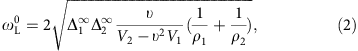where ρ1 and ρ2 denote the density of states on the two bands. Indeed, as displayed by the inset of Fig. 3, equation (2) represents an excellent parameter-free fit to the numerical data at low . For larger , on the other hand, the non-equilibrium Leggett mode deviates from the square root behaviour of equation (2). That is, as ωL approaches the Bogoliubov quasiparticle continuum, it is repelled by the lower Higgs mode ωH2, evidencing a strong coupling between them. As a result, the non-equilibrium Leggett mode is pushed below the continuum and remains nearly undamped for a wide range of , which is considerably broader than in equilibrium. Moreover, owing to the dynamical coupling among the collective modes, ωL and its higher harmonics are observable not only in the phase difference Φ1–Φ2 but also in the dynamics of the gap amplitudes Δi(t) (blue and green circles in Fig. 4).

A key advantage of measuring collective modes by pump–probe experiments is that the frequencies of the Higgs modes can be adjusted by the pump fluence. This is demonstrated in Fig. 5, which plots the dynamics of Δi(t) and Φ1–Φ2 as a function of integrated pump pulse intensity |A0|2τ. With increasing pump fluence, more Cooper pairs are broken up and superconductivity is more and more suppressed, as reflected in the reduction of the gap amplitudes. At the same time, the frequency of the Higgs oscillations decreases, as it is controlled by the superconducting gaps after pumping. Hence, it is possible to tune the lower Higgs mode ωH2 to resonance with ωL, which strongly enhances the magnitude of the collective-mode oscillations (Fig. 5a,c,e). A similar enhancement is obtained when ωH2 is brought into resonance with twice the frequency of the Leggett mode (Fig. 5b,d,f).

### Pump–probe signal

Finally, let us discuss how the Higgs and Leggett modes can be observed in pump–probe spectroscopy. In view of the recent THz pump–THz probe experiments of refs 2, 3, 4, we focus on the dynamics of the optical pump–probe conductivity σ(δt, ω)=j(δt, ω)/[iωA(δt,ω)], where δt is the delay time between pump and probe pulses, j(δt, ω) denotes the current density and A(δt, ω) represents the vector potential of the probe pulse. As the probe pulse has a weak intensity, we neglect terms of second order and higher in the probe field A(δt, ω). Similar to recent experiments2,3,4,5,6,7, we take the probe pulse to be very short with width τpr=0.15 ps and centre frequency ħωpr=5.5 meV (see Methods). With this choice, the probe pulse has a broad spectral bandwidth such that the dynamics of the superconductor is probed over a very wide frequency range.

In Fig. 6a we plot the real part of the pump–probe response Re[σ(δt, ω)] as a function of frequency for fixed δt. The non-equilibrium Leggett mode ωL and the Higgs-mode ωH2 reveal themselves in the pump–probe signal as sharp peaks. Figure 6b shows Re[σ(δt, ω)] versus delay time δt and frequency ω. Clear oscillations are seen as a function of delay time δt. These are most prominent at the frequencies of the lower Higgs and the Leggett modes, ωH2 and ωL, where σ(δt, ω) displays sharp edges as a function of ω (Fig. 6a). Fourier transforming with respect to δt shows that the dominant oscillations are ωH2 and ωL (and its higher harmonics) (Fig. 6b). We therefore predict that both the lower Higgs mode ωH2 and the Leggett mode ωL can be observed in THz pump–THz probe experiments as oscillations of the pump–probe conductivity, in particular at the gap edge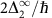and the Leggett mode frequency ωL. The higher Higgs mode ωH1, on the other hand, is not visible in the pump–probe signal, as it is strongly damped by the two-particle continuum.

## Discussion

Using a semi-numerical method based on the density matrix approach, we have studied the non-equilibrium excitation of Higgs and Leggett modes in two-band superconductors. Although the amplitude Higgs and the Leggett phase mode are decoupled in equilibrium, we find that the out-of-equilibrium excitation process leads to a strong coupling between these two collective modes. As a result, the Leggett phase mode ωL is pushed below the Bogoliubov quasiparticle continuum and remains undamped for a wide range of interband couplings (Figs 3 and 4). Likewise, the lower Higgs mode ωH2 is only weakly damped, as its frequency is at the threshold to the quasiparticle continuum. To maximize the oscillatory signal of these collective modes in the pump–probe spectra, it is necessary to choose the experimental parameters as follows: (i) the pump-pulse duration τ should be smaller than the intrinsic response time of the superconductor h/(2|Δi|), such that the collective modes are excited in a non-adiabatic manner; (ii) the pump-pulse energy needs to be of the order of the superconducting gap (that is, in the terahertz regime), so that Bogoliubov quasiparticles are excited across the gap, but modes at higher energies ħωi| are not populated; and (iii) the pump-pulse intensity must not exceed a few nJ cm−2, to ensure that the superconducting condensate is only partially broken up, but not completely destroyed. We have predicted that under these conditions both Higgs and Leggett modes can be observed as clear oscillations in the time-resolved pump–probe absorption spectra (Fig. 6). Similarly, we expect that collective mode oscillations are visible in other pump–probe-type experiments, for example, in time-resolved photoemission spectroscopy or time-resolved Raman scattering.

Our findings apply beyond the scope of two-band superconductors to general collective modes in quantum materials. That is, we expect that out-of-equilibrium excitations lead to the coupling of collective modes in any symmetry-broken phase. It would be particularly intriguing to study this in more detail for the case of unconventional exotic superconductors, where several competing orders are present, such as heavy fermion superconductors or high-temperature cuprate and pnictide superconductors. In these systems the pump pulse could be used to induce a transition from one competing order to another. Furthermore, the unconventional pairing symmetries of these superconductors, such as the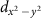-wave pairing of the cuprates, give rise to a multitude of new Higgs modes44. Our work indicates that pump–probe experiments will allow to coherently excite and control these novel Higgs modes, which await to be further explored both theoretically and experimentally.

## Methods

### Model definition

The gap equations for the BCS Hamiltonian HBCS (see equation (1) in the main text) are given by45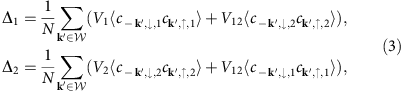where N is the number of lattice points, V1 and V2 denote the intraband interactions and V12=V1 is the interband coupling. The two-band superconductor is brought out of equilibrium via the coupling to a pump pulse, which is modelled by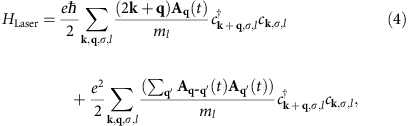where ml is the effective electron mass of the lth band and Aq(t) represents the transverse vector potential of the pump laser. We consider a Gaussian pump pulse described by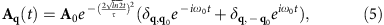with central frequency ω0, pulse width τ, light-field amplitude A0=|A0|êy and photon wave vector q0=q0êx.

### Density matrix formalism

To simulate the non-equilibrium dynamics of the two-band superconductor (1), we use a semi-numerical method based on the density matrix formalism. This approach involves the analytical derivation of equations of motions for the Bogoliubov quasiparticle densities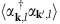,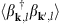,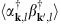and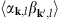, which are then integrated up numerically using a Runge Kutta algorithm. The Bogoliubov quasiparticle densities are defined in terms of the fermionic operators αk,l and βk,l, with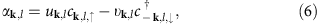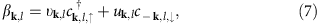where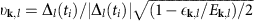,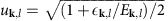and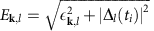. We emphasize that the coefficients uk,l and k,l do not depend on time, that is, the temporal evolution of the quasiparticle densities is computed with respect to a fixed time-independent Bogoliubov-de Gennes basis in which the initial state is diagonal. The equations of motion for the quasiparticle densities are readily obtained from Heisenberg’s equation of motion. Since equation (1) represents a mean-field Hamiltonian, this yields a closed system of differential equations and hence no truncation is needed (for details, see refs 17, 29, 23, 25).

### Pump–probe response

All physical observables, such as the current density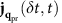, can be expressed in terms of the quasiparticle densities. For the current density we find thatwhere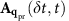and qpr=|qpr|êx are the vector potential and the wave vector of the probe pulse, respectively. With this, we obtain the pump–probe conductivity via23,46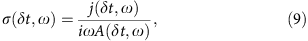where j(δt,ω) and A(δt,ω) denote the Fourier transformed y components of the current densityand the vector potential, respectively. To compute the effects of the probe pulse, we neglect terms of second order and higher in the probe field Apr(t), as the probe pulse has a very weak intensity.

### Numerical discretization and integration

To keep the number of equations of motion manageable, we have to restrict the number of considered points in momentum space. The first restriction is that we only take expectation values with indices k and k+qW into account. This means that we concentrate on the k-values where the attractive pairing interaction takes place. Furthermore, as the external electromagnetic field may add or subtract only momentum n q0, it is sufficient to consider expectation values with indices (k, k + n q0), where n. For small amplitudes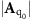the off-diagonal elements of the quasiparticle densities decrease rapidly as n increases, as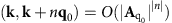. Thus, we set all entries with n>4 to 0. With this momentum–space discretization, we obtain of the order of 105 equations, which we are able to solve numerically using high-efficiency parallelization. Further, technical details can be found in refs 23, 25.

### Data availability

All relevant numerical data are available from the authors upon request.

How to cite this article: Krull, H. et al. Coupling of Higgs and Leggett modes in non-equilibrium superconductors. Nat. Commun. 7:11921 doi: 10.1038/ncomms11921 (2016).

## References

1. Leggett, A. J. Number-phase fluctuations in two-band superconductors. Prog. Theor. Phys. 36, 901–930 (1966).

2. Matsunaga, R. & Shimano, R. Nonequilibrium BCS state dynamics induced by intense terahertz pulses in a superconducting NbN film. Phys. Rev. Lett. 109, 187002 (2012).

3. Matsunaga, R. et al. Higgs amplitude mode in the BCS superconductors Nb1-xTixN induced by terahertz pulse excitation. Phys. Rev. Lett. 111, 057002 (2013).

4. Matsunaga, R. et al. Light-induced collective pseudospin precession resonating with Higgs mode in a superconductor. Science 345, 1145–1149 (2014).

5. Mansart, B. et al. Coupling of a high-energy excitation to superconducting quasiparticles in a cuprate from coherent charge fluctuation spectroscopy. Proc. Natl Acad. Sci. USA 110, 4539–4544 (2013).

6. Pashkin, A. & Leitenstorfer, A. Particle physics in a superconductor. Science 345, 1121–1122 (2014).

7. Dal Conte, S. et al. Disentangling the electronic and phononic glue in a high-Tc superconductor. Science 335, 1600–1603 (2012).

8. Beck, M. et al. Transient increase of the energy gap of superconducting nbn thin films excited by resonant narrow-band terahertz pulses. Phys. Rev. Lett. 110, 267003 (2013).

9. Pashkin, A. et al. Femtosecond response of quasiparticles and phonons in superconducting YBa2Cu3O7−x studied by wideband terahertz spectroscopy. Phys. Rev. Lett. 105, 067001 (2010).

10. Beck, M. et al. Energy-gap dynamics of superconducting NbN thin films studied by time-resolved terahertz spectroscopy. Phys. Rev. Lett. 107, 177007 (2011).

11. Hellmann, S. et al. Time-domain classification of charge-density-wave insulators. Nat. Commun. 3, 1069 (2012).

12. Torchinsky, D. H., Mahmood, F., Bollinger, A. T., Božovic′, I. & Gedik, N. Fluctuating charge-density waves in a cuprate superconductor. Nat. Mater. 12, 387–391 (2013).

13. Kimel, A. V., Kirilyuk, A., Tsvetkov, A., Pisarev, R. V. & Rasing, T Laser-induced ultrafast spin reorientation in the antiferromagnet TmFeO3 . Nature 429, 850–853 (2004).

14. Amin, M. H. S., Bezuglyi, E. V., Kijko, A. S. & Omelyanchouk, A. N. Wigner distribution function formalism for superconductors and collisionless dynamics of the superconducting order parameter. Low Temp. Phys. 30, 661–666 (2004).

15. Volkov, A. F. & Kogan, S. M. Collisionless relaxation of the energy gap in superconductors. Sov. Phys. JETP 38, 1018 (1974).

16. Schnyder, A. P., Manske, D. & Avella, A. Resonant generation of coherent phonons in a superconductor by ultrafast optical pump pulses. Phys. Rev. B 84, 214513 (2011).

17. Papenkort, T., Axt, V. M. & Kuhn, T. Coherent dynamics and pump-probe spectra of BCS superconductors. Phys. Rev. B 76, 224522 (2007).

18. Papenkort, T., Kuhn, T. & Axt, V. M. Coherent control of the gap dynamics of BCS superconductors in the nonadiabatic regime. Phys. Rev. B 78, 132505 (2008).

19. Yuzbashyan, E. A., Tsyplyatyev, O. & Altshuler, B. L. Relaxation and persistent oscillations of the order parameter in fermionic condensates. Phys. Rev. Lett. 96, 097005 (2006).

20. Yuzbashyan, E. A., Altshuler, B. L., Kuznetsov, V. B. & Enolskii, V. Z. Nonequilibrium cooper pairing in the nonadiabatic regime. Phys. Rev. B 72, 220503 (2005).

21. Yuzbashyan, E. A., Altshuler, B. L., Kuznetsov, V. B. & Enolskii, V. Z. Solution for the dynamics of the BCS and central spin problems. J. Phys. A Math. Gen. 38, 7831 (2005).

22. Zachmann, M. et al. Ultrafast terahertz-field-induced dynamics of superconducting bulk and quasi-1d samples. New J. Phys. 15, 055016 (2013).

23. Krull, H., Manske, D., Uhrig, G. S. & Schnyder, A. P. Signatures of nonadiabatic BCS state dynamics in pump-probe conductivity. Phys. Rev. B 90, 014515 (2014).

24. Yuzbashyan, E. A., Dzero, M., Gurarie, V. & Foster, M. S. Quantum quench phase diagrams of an s-wave BCS -BEC condensate. Phys. Rev. A 91, 033628 (2015).

25. Krull, H. Conductivity of Strongly Pumped Superconductors. PhD thesis, TU Dortmund (2014).

26. Kemper, A. F., Sentef, M. A., Moritz, B., Freericks, J. K. & Devereaux, T. P. Direct observation of Higgs mode oscillations in the pump-probe photoemission spectra of electron-phonon mediated superconductors. Phys. Rev. B 92, 224517 (2015).

27. Peronaci, F., Schiró, M. & Capone, M. Transient dynamics of d-wave superconductors after a sudden excitation. Phys. Rev. Lett. 115, 257001 (2015).

28. Murakami, Y., Werner, P., Tsuji, N. & Aoki, H. Multiple amplitude modes in strongly coupled phonon-mediated superconductors. Phys. Rev. B 93, 094509 (2016).

29. Akbari, A., Schnyder, A. P., Manske, D. & Eremin, I. Theory of nonequilibrium dynamics of multiband superconductors. Europhys. Lett. 101, 17002 (2013).

30. Dzero, M., Khodas, M. & Levchenko, A. Amplitude modes and dynamic coexistence of competing orders in multicomponent superconductors. Phys. Rev. B 91, 214505 (2015).

31. Demsar, J. et al. Pair-breaking and superconducting state recovery dynamics inMgB2 . Phys. Rev. Lett. 91, 267002 (2003).

32. Burnell, F. J., Hu, J., Parish, M. M. & Bernevig, B. A. Leggett mode in a strong-coupling model of iron arsenide superconductors. Phys. Rev. B 82, 144506 (2010).

33. Anishchanka, A., Volkov, A. F. & Efetov, K. B. Collective modes in two-band superconductors in the dirty limit. Phys. Rev. B 76, 104504 (2007).

34. Pekker, D. & Varma, C. Amplitude/Higgs modes in condensed matter physics. Annu. Rev. Condensed Matter Phys. 6, 269–297 (2015).

35. Anderson, P. W. Random-phase approximation in the theory of superconductivity. Phys. Rev. 112, 1900–1916 (1958).

36. Bogoliubov, N. N., Tolmatschev, V. V. & Shirkov, D. V. New Method in the Theory of Superconductivity Publisher Consultants Bureau: New York, (1959).

37. Podolsky, D., Auerbach, A. & Arovas, D. P. Visibility of the amplitude (Higgs) mode in condensed matter. Phys. Rev. B 84, 174522 (2011).

38. Sooryakumar, R. & Klein, M. V. Raman scattering by superconducting-gap excitations and their coupling to charge-density waves. Phys. Rev. Lett. 45, 660–662 (1980).

39. Littlewood, P. B. & Varma, C. M. Gauge-invariant theory of the dynamical interaction of charge density waves and superconductivity. Phys. Rev. Lett. 47, 811–814 (1981).

40. Blumberg, G. et al. Observation of Leggett’s collective mode in a multiband MgB2 superconductor. Phys. Rev. Lett. 99, 227002 (2007).

41. Méasson, M.-A. et al. Amplitude Higgs mode in the 2H-NbSe2 superconductor. Phys. Rev. B 89, 060503 (2014).

42. Mazin, I. & Antropov, V. Electronic structure, electron–phonon coupling, and multiband effects in MgB2 . Phys. C Superconductivity 385, 49–65 (2003).

43. Sharapov, S. G., Gusynin, V. P. & Beck, H. Effective action approach to the Leggett’s mode in two-band superconductors. Eur. Phys. J. B 30, 45–51 (2002).

44. Barlas, Y. & Varma, C. M. Amplitude or Higgs modes in d-wave superconductors. Phys. Rev. B 87, 054503 (2013).

45. Suhl, H., Matthias, B. T. & Walker, L. R. Bardeen-Cooper-Schrieffer theory of superconductivity in the case of overlapping bands. Phys. Rev. Lett. 3, 552–554 (1959).

46. Shao, C., Tohyama, T., Luo, H.-G. & Lu, H. A numerical method to compute optical conductivity based on the pump-probe simulations. Preprint at http://arXiv.org/abs/1507.01200 (2015).

## Acknowledgements

We gratefully acknowledge many useful discussions with A. Avella, S. Kaiser and R. Shimano. G.S.U. and H.K. acknowledge financial support by the DFG in TRR 160 and by the Stiftung Mercator. H.K. thanks the Max-Planck-Institut FKF Stuttgart for its hospitality.

## Author information

Authors

### Contributions

The density matrix simulations were developed and run by H.K., N.B. and A.P.S. All authors contributed to the discussion and interpretation of the results, and to the writing of the paper.

### Corresponding author

Correspondence to A. P. Schnyder.

## Ethics declarations

### Competing interests

The authors declare no competing financial interests.

## Rights and permissions

Reprints and Permissions

• ### Visualization and quantum control of light-accelerated condensates by terahertz multi-dimensional coherent spectroscopy

• Martin Mootz
• Liang Luo
• llias E. Perakis

Communications Physics (2022)

• ### Spectroscopic signatures of time-reversal symmetry breaking superconductivity

• Nicholas R. Poniatowski
• Jonathan B. Curtis
• Prineha Narang

Communications Physics (2022)

• ### Light quantum control of persisting Higgs modes in iron-based superconductors

• C. Vaswani
• J. H. Kang
• J. Wang

Nature Communications (2021)

• ### Classification and characterization of nonequilibrium Higgs modes in unconventional superconductors

• L. Schwarz
• B. Fauseweh
• D. Manske

Nature Communications (2020)

• ### Non-equilibrium Phenomena in Superconductors Probed by Femtosecond Time-Domain Spectroscopy

• J. Demsar

Journal of Low Temperature Physics (2020)